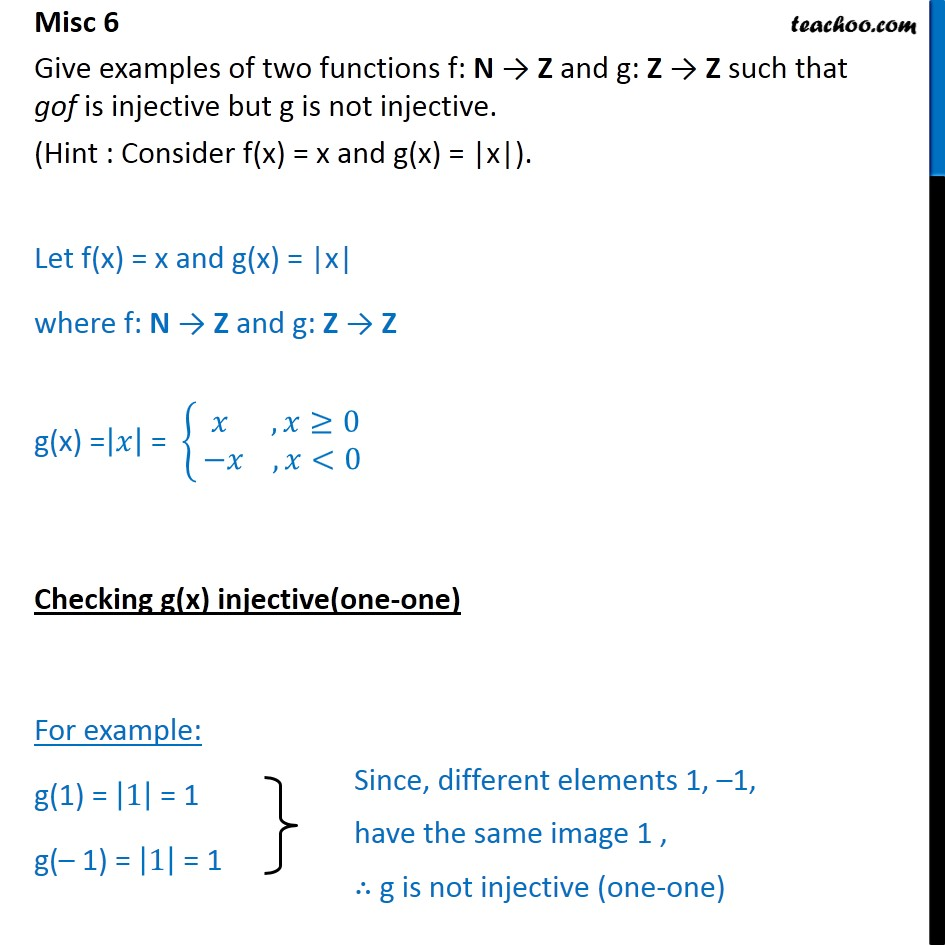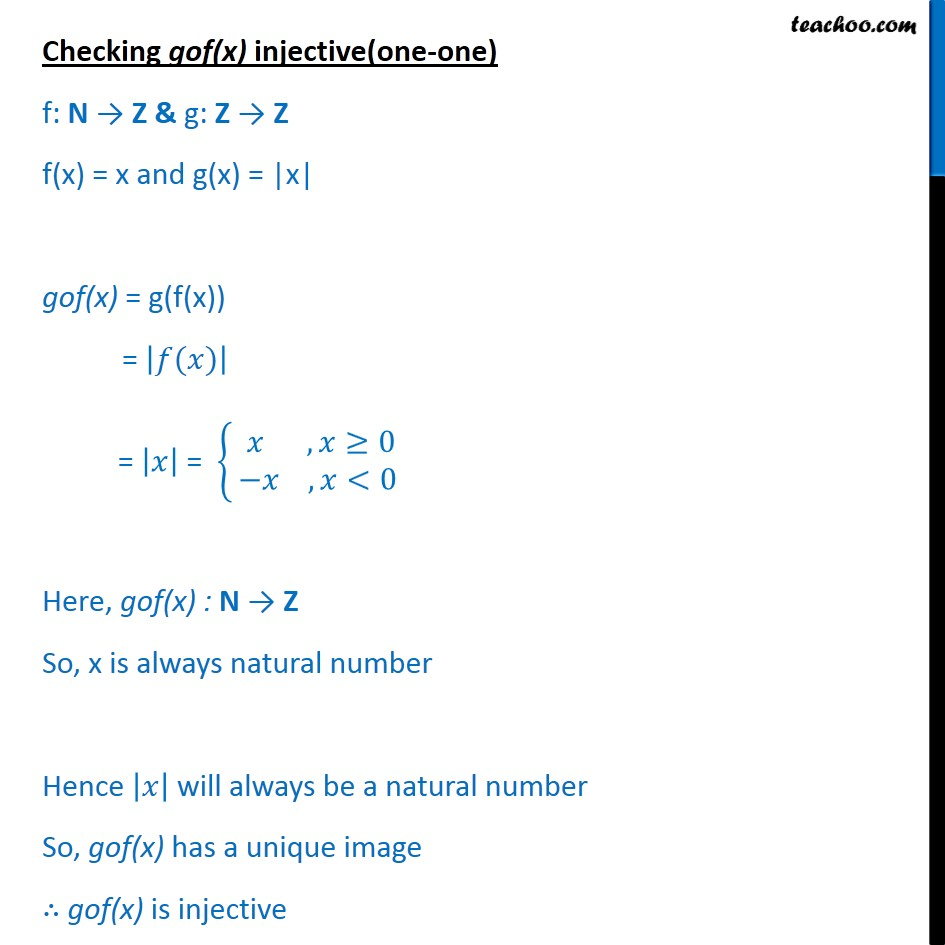Miscellaneous

Chapter 1 Class 12 Relation and Functions (Term 1)
Serial order wise### Transcript

Misc 6 Give examples of two functions f: N → Z and g: Z → Z such that gof is injective but g is not injective. (Hint : Consider f(x) = x and g(x) = |x|). Let f(x) = x and g(x) = |x| where f: N → Z and g: Z → Z g(x) = 𝑥﷯ = 𝑥 , 𝑥≥0 ﷮−𝑥 , 𝑥<0﷯﷯ Checking g(x) injective(one-one) For example: g(1) = 1﷯ = 1 g(– 1) = 1﷯ = 1 Checking gof(x) injective(one-one) f: N → Z & g: Z → Z f(x) = x and g(x) = |x| gof(x) = g(f(x)) = 𝑓(𝑥)﷯ = 𝑥﷯ = 𝑥 , 𝑥≥0 ﷮−𝑥 , 𝑥<0﷯﷯ Here, gof(x) : N → Z So, x is always natural number Hence 𝑥﷯ will always be a natural number So, gof(x) has a unique image ∴ gof(x) is injective